login  home  contents  what's new  discussion  bug reports help  links  subscribe  changes  refresh  edit

# Edit detail for #347 bug in map$Set revision 1 of 1  1 Editor: Time: 2007/11/17 22:30:51 GMT-8 Note: fixed in wh-sandbox revision 489 changed: - map confuses sets. \begin{axiom} A:Set Integer:=set [-2,-1,0] B:Set Integer:=set [0,1,4] C:=map(x +-> x^2,A) test(C=B) \end{axiom} somehow a sort is missing after applying map. Proposed Fix If 'S has OrderedSet' then 'map_!' should include 'sort':: map_!(f,s) == map_!(f,s)$Rep
sort_!(s)$Rep removeDuplicates_! See "diff":/axiom--test--1/src/algebra/SetsSpad/diff See also: SandBoxSetAny for a more ambitious fix that defines an ordering for all Sets. \begin{spad} )abbrev domain SET Set ++ Author: Michael Monagan; revised by Richard Jenks ++ Date Created: August 87 through August 88 ++ Date Last Updated: May 1991 ++ Basic Operations: ++ Related Constructors: ++ Also See: ++ AMS Classifications: ++ Keywords: ++ References: ++ Description: ++ A set over a domain D models the usual mathematical notion of a finite set ++ of elements from D. ++ Sets are unordered collections of distinct elements ++ (that is, order and duplication does not matter). ++ The notation \spad{set [a,b,c]} can be used to create ++ a set and the usual operations such as union and intersection are available ++ to form new sets. ++ In our implementation, \Language{} maintains the entries in ++ sorted order. Specifically, the parts function returns the entries ++ as a list in ascending order and ++ the extract operation returns the maximum entry. ++ Given two sets s and t where \spad{#s = m} and \spad{#t = n}, ++ the complexity of ++ \spad{s = t} is \spad{O(min(n,m))} ++ \spad{s < t} is \spad{O(max(n,m))} ++ \spad{union(s,t)}, \spad{intersect(s,t)}, \spad{minus(s,t)}, \spad{symmetricDifference(s,t)} is \spad{O(max(n,m))} ++ \spad{member(x,t)} is \spad{O(n log n)} ++ \spad{insert(x,t)} and \spad{remove(x,t)} is \spad{O(n)} Set(S:SetCategory): FiniteSetAggregate S == add Rep := FlexibleArray(S) # s == _#$Rep s
brace()   == empty()
set()     == empty()
empty()   == empty()$Rep copy s == copy(s)$Rep
parts s   == parts(s)$Rep inspect s == (empty? s => error "Empty set"; s(maxIndex s)) extract_! s == x := inspect s delete_!(s, maxIndex s) x find(f, s) == find(f, s)$Rep

map(f, s) == map_!(f,copy s)

reduce(f, s) == reduce(f, s)$Rep reduce(f, s, x) == reduce(f, s, x)$Rep

reduce(f, s, x, y) == reduce(f, s, x, y)$Rep if S has ConvertibleTo InputForm then convert(x:%):InputForm == convert [convert("set"::Symbol)@InputForm, convert(parts x)@InputForm] if S has OrderedSet then s = t == s =$Rep t
max s == inspect s
min s == (empty? s => error "Empty set"; s(minIndex s))

map_!(f,s) ==
map_!(f,s)$Rep sort_!(s)$Rep
removeDuplicates_! s

construct l ==
zero?(n := #l) => empty()
a := new(n, first l)
for i in minIndex(a).. for x in l repeat a.i := x
removeDuplicates_! sort_! a

insert_!(x, s) ==
n := inc maxIndex s
k := minIndex s
while k < n and x > s.k repeat k := inc k
k < n and s.k = x => s
insert_!(x, s, k)

member?(x, s) == -- binary search
empty? s => false
t := maxIndex s
b := minIndex s
while b < t repeat
m := (b+t) quo 2
if x > s.m then b := m+1 else t := m
x = s.t

remove_!(x:S, s:%) ==
n := inc maxIndex s
k := minIndex s
while k < n and x > s.k repeat k := inc k
k < n and x = s.k => delete_!(s, k)
s

-- the set operations are implemented as variations of merging
intersect(s, t) ==
m := maxIndex s
n := maxIndex t
i := minIndex s
j := minIndex t
r := empty()
while i <= m and j <= n repeat
s.i = t.j => (concat_!(r, s.i); i := i+1; j := j+1)
if s.i < t.j then i := i+1 else j := j+1
r

difference(s:%, t:%) ==
m := maxIndex s
n := maxIndex t
i := minIndex s
j := minIndex t
r := empty()
while i <= m and j <= n repeat
s.i = t.j => (i := i+1; j := j+1)
s.i < t.j => (concat_!(r, s.i); i := i+1)
j := j+1
while i <= m repeat (concat_!(r, s.i); i := i+1)
r

symmetricDifference(s, t) ==
m := maxIndex s
n := maxIndex t
i := minIndex s
j := minIndex t
r := empty()
while i <= m and j <= n repeat
s.i < t.j => (concat_!(r, s.i); i := i+1)
s.i > t.j => (concat_!(r, t.j); j := j+1)
i := i+1; j := j+1
while i <= m repeat (concat_!(r, s.i); i := i+1)
while j <= n repeat (concat_!(r, t.j); j := j+1)
r

subset?(s, t) ==
m := maxIndex s
n := maxIndex t
m > n => false
i := minIndex s
j := minIndex t
while i <= m and j <= n repeat
s.i = t.j => (i := i+1; j := j+1)
s.i > t.j => j := j+1
return false
i > m

union(s:%, t:%) ==
m := maxIndex s
n := maxIndex t
i := minIndex s
j := minIndex t
r := empty()
while i <= m and j <= n repeat
s.i = t.j => (concat_!(r, s.i); i := i+1; j := j+1)
s.i < t.j => (concat_!(r, s.i); i := i+1)
(concat_!(r, t.j); j := j+1)
while i <= m repeat (concat_!(r, s.i); i := i+1)
while j <= n repeat (concat_!(r, t.j); j := j+1)
r

else
map_!(f,s) ==
map_!(f,s)$Rep removeDuplicates_! s insert_!(x, s) == for k in minIndex s .. maxIndex s repeat s.k = x => return s insert_!(x, s, inc maxIndex s) remove_!(x:S, s:%) == n := inc maxIndex s k := minIndex s while k < n repeat x = s.k => return delete_!(s, k) k := inc k s \end{spad} Retest \begin{axiom} A2:Set Integer:=set [-2,-1,0] B2:Set Integer:=set [0,1,4] C2:=map(x +-> x^2,A) test(B2=C2) \end{axiom} But unfortunately the documentation lies:: ++ In our implementation, \Language{} maintains the entries in ++ sorted order. Specifically, the parts function returns the ++ entries as a list in ascending order and the extract operation ++ returns the maximum entry. This example shows that Set is not maintained in sorterd order. So the code for Set still appears to be broken if the Set is constructed over a domain that is not an OrderedSet. \begin{axiom} )set message any off showTypeInOutput true; \end{axiom} \begin{axiom} Set Any has OrderedSet B3:Set Any:=B;B3 C3:Set Any:=C;C3 test(B3=C3) \end{axiom} But why does this example work? Is set equality implemented as an order n^2 comparison if the domain is not an OrderedSet? From BillPage Thu Apr 5 15:26:15 -0500 2007 From: Bill Page Date: Thu, 05 Apr 2007 15:26:15 -0500 Subject: Re: order n^2 comparison, answer: yes Message-ID: <20070405152615-0500@wiki.axiom-developer.org> In FiniteSetAggregate we see:: s = t == #s = #t and empty? difference(s,t) ... difference(s:%, t:%) == m := copy s for x in parts t repeat remove_!(x, m) m From BillPage Mon Apr 9 00:49:11 -0500 2007 From: Bill Page Date: Mon, 09 Apr 2007 00:49:11 -0500 Subject: fixed in wh-sandbox revision 489 Message-ID: <20070409004911-0500@wiki.axiom-developer.org> Status: open => fix proposed   Submitted by : (unknown) at: 2007-11-17T22:30:51-08:00 (15 years ago) Name : Axiom Version : default friCAS-20090114 Axiom-20050901 OpenAxiom-20091012 OpenAxiom-20110220 OpenAxiom-Release-141 Category : Axiom Aldor Interface Axiom Compiler Axiom Library Axiom Interpreter Axiom Documentation Axiom User Interface building Axiom from source lisp system MathAction Doyen CD Reduce Axiom on Windows Axiom on Linux Severity : critical serious normal minor wishlist Status : open closed rejected not reproducible fix proposed fixed somewhere duplicate need more info Optional subject : Optional comment : map confuses sets. axiom A:Set Integer:=set [-2,-1,0]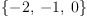(1) Type: Set(Integer) axiom B:Set Integer:=set [0,1,4]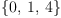(2) Type: Set(Integer) axiom C:=map(x +-> x^2,A)(3) Type: Set(Integer) axiom test(C=B)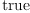(4) Type: Boolean somehow a sort is missing after applying map. ## Proposed Fix If S has OrderedSet then map_! should include 'sort':  map_!(f,s) == map_!(f,s)$Rep
sort_!(s)$Rep removeDuplicates_!  See diff See also: SandBoxSetAny for a more ambitious fix that defines an ordering for all Sets. spad )abbrev domain SET Set ++ Author: Michael Monagan; revised by Richard Jenks ++ Date Created: August 87 through August 88 ++ Date Last Updated: May 1991 ++ Basic Operations: ++ Related Constructors: ++ Also See: ++ AMS Classifications: ++ Keywords: ++ References: ++ Description: ++ A set over a domain D models the usual mathematical notion of a finite set ++ of elements from D. ++ Sets are unordered collections of distinct elements ++ (that is, order and duplication does not matter). ++ The notation \spad{set [a,b,c]} can be used to create ++ a set and the usual operations such as union and intersection are available ++ to form new sets. ++ In our implementation, \Language{} maintains the entries in ++ sorted order. Specifically, the parts function returns the entries ++ as a list in ascending order and ++ the extract operation returns the maximum entry. ++ Given two sets s and t where \spad{#s = m} and \spad{#t = n}, ++ the complexity of ++ \spad{s = t} is \spad{O(min(n,m))} ++ \spad{s < t} is \spad{O(max(n,m))} ++ \spad{union(s,t)}, \spad{intersect(s,t)}, \spad{minus(s,t)}, \spad{symmetricDifference(s,t)} is \spad{O(max(n,m))} ++ \spad{member(x,t)} is \spad{O(n log n)} ++ \spad{insert(x,t)} and \spad{remove(x,t)} is \spad{O(n)} Set(S:SetCategory): FiniteSetAggregate S == add Rep := FlexibleArray(S) # s == _#$Rep s
brace()   == empty()
set()     == empty()
empty()   == empty()$Rep copy s == copy(s)$Rep
parts s   == parts(s)$Rep inspect s == (empty? s => error "Empty set"; s(maxIndex s)) extract_! s == x := inspect s delete_!(s, maxIndex s) x find(f, s) == find(f, s)$Rep
map(f, s) == map_!(f,copy s)
reduce(f, s) == reduce(f, s)$Rep reduce(f, s, x) == reduce(f, s, x)$Rep
reduce(f, s, x, y) == reduce(f, s, x, y)$Rep if S has ConvertibleTo InputForm then convert(x:%):InputForm == convert [convert("set"::Symbol)@InputForm, convert(parts x)@InputForm] if S has OrderedSet then s = t == s =$Rep t
max s == inspect s
min s == (empty? s => error "Empty set"; s(minIndex s))
map_!(f,s) ==
map_!(f,s)$Rep sort_!(s)$Rep
removeDuplicates_! s
construct l ==
zero?(n := #l) => empty()
a := new(n, first l)
for i in minIndex(a).. for x in l repeat a.i := x
removeDuplicates_! sort_! a
insert_!(x, s) ==
n := inc maxIndex s
k := minIndex s
while k < n and x > s.k repeat k := inc k
k < n and s.k = x => s
insert_!(x, s, k)
member?(x, s) == -- binary search
empty? s => false
t := maxIndex s
b := minIndex s
while b < t repeat
m := (b+t) quo 2
if x > s.m then b := m+1 else t := m
x = s.t
remove_!(x:S, s:%) ==
n := inc maxIndex s
k := minIndex s
while k < n and x > s.k repeat k := inc k
k < n and x = s.k => delete_!(s, k)
s
-- the set operations are implemented as variations of merging
intersect(s, t) ==
m := maxIndex s
n := maxIndex t
i := minIndex s
j := minIndex t
r := empty()
while i <= m and j <= n repeat
s.i = t.j => (concat_!(r, s.i); i := i+1; j := j+1)
if s.i < t.j then i := i+1 else j := j+1
r
difference(s:%, t:%) ==
m := maxIndex s
n := maxIndex t
i := minIndex s
j := minIndex t
r := empty()
while i <= m and j <= n repeat
s.i = t.j => (i := i+1; j := j+1)
s.i < t.j => (concat_!(r, s.i); i := i+1)
j := j+1
while i <= m repeat (concat_!(r, s.i); i := i+1)
r
symmetricDifference(s, t) ==
m := maxIndex s
n := maxIndex t
i := minIndex s
j := minIndex t
r := empty()
while i <= m and j <= n repeat
s.i < t.j => (concat_!(r, s.i); i := i+1)
s.i > t.j => (concat_!(r, t.j); j := j+1)
i := i+1; j := j+1
while i <= m repeat (concat_!(r, s.i); i := i+1)
while j <= n repeat (concat_!(r, t.j); j := j+1)
r
subset?(s, t) ==
m := maxIndex s
n := maxIndex t
m > n => false
i := minIndex s
j := minIndex t
while i <= m and j <= n repeat
s.i = t.j => (i := i+1; j := j+1)
s.i > t.j => j := j+1
return false
i > m
union(s:%, t:%) ==
m := maxIndex s
n := maxIndex t
i := minIndex s
j := minIndex t
r := empty()
while i <= m and j <= n repeat
s.i = t.j => (concat_!(r, s.i); i := i+1; j := j+1)
s.i < t.j => (concat_!(r, s.i); i := i+1)
(concat_!(r, t.j); j := j+1)
while i <= m repeat (concat_!(r, s.i); i := i+1)
while j <= n repeat (concat_!(r, t.j); j := j+1)
r
else
map_!(f,s) ==
map_!(f,s)$Rep removeDuplicates_! s insert_!(x, s) == for k in minIndex s .. maxIndex s repeat s.k = x => return s insert_!(x, s, inc maxIndex s) remove_!(x:S, s:%) == n := inc maxIndex s k := minIndex s while k < n repeat x = s.k => return delete_!(s, k) k := inc k s spad  Compiling FriCAS source code from file /var/zope2/var/LatexWiki/7072998645375249993-25px002.spad using old system compiler. SET abbreviates domain Set ------------------------------------------------------------------------ initializing NRLIB SET for Set compiling into NRLIB SET compiling exported # :$ -> NonNegativeInteger
;;;     ***       |SET;#;$Nni;1| REDEFINED Time: 0.28 SEC. compiling exported brace : () ->$
;;;     ***       |SET;brace;$;2| REDEFINED Time: 0 SEC. compiling exported set : () ->$
;;;     ***       |SET;set;$;3| REDEFINED Time: 0 SEC. compiling exported empty : () ->$
;;;     ***       |SET;empty;$;4| REDEFINED Time: 0 SEC. compiling exported copy :$ -> $;;; *** |SET;copy;2$;5| REDEFINED
Time: 0 SEC.
compiling exported parts : $-> List S ;;; *** |SET;parts;$L;6| REDEFINED
Time: 0 SEC.
compiling exported inspect : $-> S ;;; *** |SET;inspect;$S;7| REDEFINED
Time: 0.06 SEC.
compiling exported extract! : $-> S ;;; *** |SET;extract!;$S;8| REDEFINED
Time: 0 SEC.
compiling exported find : (S -> Boolean,$) -> Union(S,failed) ;;; *** |SET;find;M$U;9| REDEFINED
Time: 0 SEC.
compiling exported map : (S -> S,$) ->$
;;;     ***       |SET;map;M2$;10| REDEFINED Time: 0 SEC. compiling exported reduce : ((S,S) -> S,$) -> S
;;;     ***       |SET;reduce;M$S;11| REDEFINED Time: 0.01 SEC. compiling exported reduce : ((S,S) -> S,$,S) -> S
;;;     ***       |SET;reduce;M$2S;12| REDEFINED Time: 0 SEC. compiling exported reduce : ((S,S) -> S,$,S,S) -> S
;;;     ***       |SET;reduce;M$3S;13| REDEFINED Time: 0 SEC. ****** Domain: S already in scope augmenting S: (ConvertibleTo (InputForm)) compiling exported convert :$ -> InputForm
;;;     ***       |SET;convert;$If;14| REDEFINED Time: 0.28 SEC. ****** Domain: S already in scope augmenting S: (OrderedSet) compiling exported = : ($,$) -> Boolean ;;; *** |SET;=;2$B;15| REDEFINED
Time: 0.01 SEC.
compiling exported max : $-> S ;;; *** |SET;max;$S;16| REDEFINED
Time: 0 SEC.
compiling exported min : $-> S ;;; *** |SET;min;$S;17| REDEFINED
Time: 0 SEC.
compiling exported map! : (S -> S,$) ->$
;;;     ***       |SET;map!;M2$;18| REDEFINED Time: 0.01 SEC. compiling exported construct : List S ->$
;;;     ***       |SET;construct;L$;19| REDEFINED Time: 0.01 SEC. compiling exported insert! : (S,$) -> $;;; *** |SET;insert!;S2$;20| REDEFINED
Time: 0.01 SEC.
compiling exported member? : (S,$) -> Boolean ;;; *** |SET;member?;S$B;21| REDEFINED
Time: 0.01 SEC.
compiling exported remove! : (S,$) ->$
;;;     ***       |SET;remove!;S2$;22| REDEFINED Time: 0.01 SEC. compiling exported intersect : ($,$) ->$
;;;     ***       |SET;intersect;3$;23| REDEFINED Time: 0 SEC. compiling exported difference : ($,$) ->$
;;;     ***       |SET;difference;3$;24| REDEFINED Time: 0 SEC. compiling exported symmetricDifference : ($,$) ->$
;;;     ***       |SET;symmetricDifference;3$;25| REDEFINED Time: 0 SEC. compiling exported subset? : ($,$) -> Boolean ;;; *** |SET;subset?;2$B;26| REDEFINED
Time: 0.15 SEC.
compiling exported union : ($,$) -> $;;; *** |SET;union;3$;27| REDEFINED
Time: 0.02 SEC.
compiling exported map! : (S -> S,$) ->$
Time: 0.01 SEC.
compiling exported insert! : (S,$) ->$
Time: 0.01 SEC.
compiling exported remove! : (S,$) ->$
Time: 0.02 SEC.
****** Domain: S already in scope
augmenting S: (Evalable S)
****** Domain: S already in scope
augmenting S: (ConvertibleTo (InputForm))
****** Domain: S already in scope
augmenting S: (Finite)
****** Domain: S already in scope
augmenting S: (OrderedSet)
(time taken in buildFunctor:  10)
;;;     ***       |Set| REDEFINED
;;;     ***       |Set| REDEFINED
Time: 0.01 SEC.
Cumulative Statistics for Constructor Set
Time: 0.91 seconds
finalizing NRLIB SET
Processing Set for Browser database:
--------constructor---------
; compiling file "/var/zope2/var/LatexWiki/SET.NRLIB/SET.lsp" (written 01 AUG 2011 08:06:27 AM):
; /var/zope2/var/LatexWiki/SET.NRLIB/SET.fasl written
; compilation finished in 0:00:00.532
------------------------------------------------------------------------
Set is now explicitly exposed in frame initial
Set will be automatically loaded when needed from
/var/zope2/var/LatexWiki/SET.NRLIB/SET
>> System error:
The bounding indices 163 and 162 are bad for a sequence of length 162.
The ANSI Standard, Glossary entry for "bounding index designator"
The ANSI Standard, writeup for Issue SUBSEQ-OUT-OF-BOUNDS:IS-AN-ERROR

Retest

axiom
A2:Set Integer:=set [-2,-1,0](5)
Type: Set(Integer)
axiom
B2:Set Integer:=set [0,1,4](6)
Type: Set(Integer)
axiom
C2:=map(x +-> x^2,A)(7)
Type: Set(Integer)
axiom
test(B2=C2)(8)
Type: Boolean

But unfortunately the documentation lies:

  ++ In our implementation, \Language{} maintains the entries in
++ sorted order.  Specifically, the parts function returns the
++ entries as a list in ascending order and the extract operation
++ returns the maximum entry.


This example shows that Set is not maintained in sorterd order. So the code for Set still appears to be broken if the Set is constructed over a domain that is not an OrderedSet.

axiom
)set message any off
showTypeInOutput true;
Type: String

axiom
Set Any has OrderedSet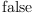(9)
Type: Boolean
axiom
B3:Set Any:=B;B3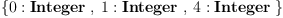(10)
Type: Set(Any)
axiom
C3:Set Any:=C;C3(11)
Type: Set(Any)
axiom
test(B3=C3)(12)
Type: Boolean

But why does this example work? Is set equality implemented as an order n^2 comparison if the domain is not an OrderedSet?

Re: order n^2 comparison, answer: yes --Bill Page, Thu, 05 Apr 2007 15:26:15 -0500 reply
In FiniteSetAggregate? we see:
   s = t           == #s = #t and empty? difference(s,t)

...

difference(s:%, t:%) ==
m := copy s
for x in parts t repeat remove_!(x, m)
m


fixed in wh-sandbox revision 489 --Bill Page, Mon, 09 Apr 2007 00:49:11 -0500 reply
Status: open => fix proposed